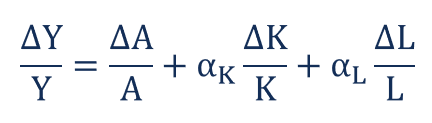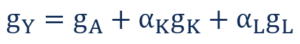# Growth Accounting Equation

Measures how changes in real GDP in an economy are influenced by changes in available capital, labor, human capital, and technology

## What is the Growth Accounting Equation?

The Growth Accounting Equation is a financial tool that measures economic growth – specifically, how changes in real Gross Domestic Product (GDP) in an economy are influenced by changes in available capital, labor, and technology. The Growth Accounting Equation facilitates analyzing economic growth at the minutest level. It enables one to break down economic growth into various components at the micro level and, thus, gives a very accurate measure of the economic growth of a nation.

### Formula

The Growth Accounting Equation is calculated as follows:Where:

• Y is GDP or total gross domestic output
• A is Total Factor Productivity
• K is the country’s existing capital stock
• L is the size of the country’s labor force
• ΔY is the change in GDP
• ΔA is the change in Total Factor Productivity
• ΔK is the change in the country’s capital stock
• ΔL is the change in the size of the country’ labor force
• αK is capital’s share of total income
• αL is labor’s share of total income

The Growth Accounting Equation can alternatively be written as follows:Where:

• gY is GDP growth
• gA is TFP (Total Factor Productivity) growth
• gK is capital stock growth
• gL is labor force growth or population growth

Here, growth rates can be positive or negative. Hence, the equation can also be used to analyze a decline in real GDP as well as an increase in real GDP. However, one problem with the formula is that the growth rate of technology is very difficult to accurately measure. Its calculation generally depends on the discretion of the accountant.

### Capital to Labor Ratio

In the growth accounting equation, the component “(change in k)/k” represents the capital to labor ratio. The capital to labor ratio is the ratio of total capital available per one unit of labor. The ratio indicates the extent of capital intensiveness (commonly referred to as capital deepening) of an economy. It shows if the economy is capital-intensive, which basically means the use of more capital and less labor, or labor-intensive, which means the use of more hands-on labor, and less capital. Usually, developed countries, or countries with prosperous economic growth, are more capital-intensive, whereas poor, or developing countries, are generally more labor-intensive.

### Impact of Capital to Labor Ratio on the Growth Accounting Equation

The capital to labor ratio indicates that the standard of living in an economy depends on the availability of capital and advancements in technology, and not just on the percentage increase in employment.

### More Resources

CFI is the official provider of the global Financial Modeling & Valuation Analyst (FMVA)™ certification program, designed to help anyone become a world-class financial analyst. To keep advancing your career, the additional CFI resources below will be useful:

• Consumer Surplus Formula
• Economic Indicators
• Gross National Product
• J Curve

### Financial Analyst Certification

Become a certified Financial Modeling and Valuation Analyst (FMVA)® by completing CFI’s online financial modeling classes!